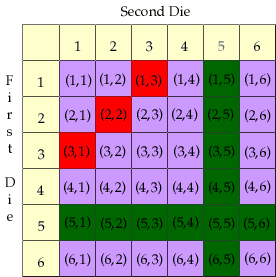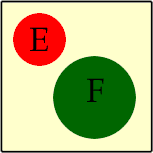Name: Marje Who is asking: Student Level: All Question: What does the mathmatical term "mutually exclusive" mean. Pleas diagram if possible. Many thanks Hi Marje, Mutually exclusive is a term used in probability and statistics. The definition is Two events E anf F are mutually exclusive if they have no outcomes in common. To use the set theory language Two events E and F are mutually exclusive if the intersection of E and F is empty. An equivalent term is disjoint. For example, suppose that you are rolling two dice. I win if the sum on the two dice is 4. You win if at least one of the dice shows a 5. These are two events. "the sum on the dice is 4" and "at least one of the dice is 5". These two events are mutually exclusive since, on one roll of the dice it is impossible for us both to win. There is no outcome that satisfies both events. Below is a diagram of that illustrates this example. I have listed all 36 possible outcomes for rolling two dice. (The list of all possible outcomes is called the sample space.) The format is (1st die,2nd die). The outcomes in the event "at least one of the dice is 5" are coloured green and the outcomes in the event "the sum on the dice is 4" are coloured red. These events are mutually exclusive since there are no events coloured both red and green.This is not the usual diagram. The usual diagram is a Venn Diagram. Draw a rectangle to represent the sample space, that is all possible outcomes. Draw a circle to surround all the outcomes in event E and another circle to surround the outcomes in event F. The events E and F are mutually exclusive if the circles do not overlap.Cheers, Penny Go to Math Central